$$\newcommand{\esp}{\mathbb{E}\left(#1\right)} \newcommand{\var}{\mbox{Var}\left(#1\right)} \newcommand{\deriv}{\dot{#1}(t)} \newcommand{\prob}{ \mathbb{P}\!(#1)} \newcommand{\eqdef}{\mathop{=}\limits^{\mathrm{def}}} \newcommand{\by}{\boldsymbol{y}} \newcommand{\bc}{\boldsymbol{c}} \newcommand{\bpsi}{\boldsymbol{\psi}} \def\pmacro{\texttt{p}} \def\like{{\cal L}} \def\llike{{\cal LL}} \def\logit{{\rm logit}} \def\probit{{\rm probit}} \def\one{{\rm 1\!I}} \def\iid{\mathop{\sim}_{\rm i.i.d.}} \def\simh0{\mathop{\sim}_{H_0}} \def\df{\texttt{df}} \def\res{e} \def\xomega{x} \newcommand{\argmin}{{\rm arg}\min_{#1}} \newcommand{\argmax}{{\rm arg}\max_{#1}} \newcommand{\Rset}{\mbox{\mathbb{R}}} \def\param{\theta} \def\setparam{\Theta} \def\xnew{x_{\rm new}} \def\fnew{f_{\rm new}} \def\ynew{y_{\rm new}} \def\nnew{n_{\rm new}} \def\enew{e_{\rm new}} \def\Xnew{X_{\rm new}} \def\hfnew{\widehat{\fnew}} \def\degree{m} \def\nbeta{d} \newcommand{\limite}{\mathop{\longrightarrow}\limits_{#1}} \def\ka{k{a}} \def\ska{k{\scriptscriptstyle a}} \def\kel{k{e}} \def\skel{k{\scriptscriptstyle e}} \def\cl{C{\small l}} \def\Tlag{T\hspace{-0.1em}{lag}} \def\sTlag{T\hspace{-0.07em}{\scriptscriptstyle lag}} \def\Tk{T\hspace{-0.1em}{k0}} \def\sTk{T\hspace{-0.07em}{\scriptscriptstyle k0}} \def\thalf{t{1/2}} \newcommand{\Dphi}{\partial_\pphi #1} \def\asigma{a} \def\pphi{\psi} \newcommand{\stheta}{{\theta^\star}} \newcommand{\htheta}{{\widehat{\theta}}}$$

# 1 Introduction

The data cars give the speed of cars and the distances taken to stop (Note that the data were recorded in the 1920s).

The data frame consists of 50 observations (rows) and 2 variables (columns): speed (mph), stopping distance (ft).

Let us plot this data.

library(ggplot2)
theme_set(theme_bw())
pl <- ggplot(cars, aes(x=speed, y=dist)) + geom_point(size=2, colour="#993399") +
xlab("Speed (mph)") + ylab("Stopping distance (ft)")
print(pl)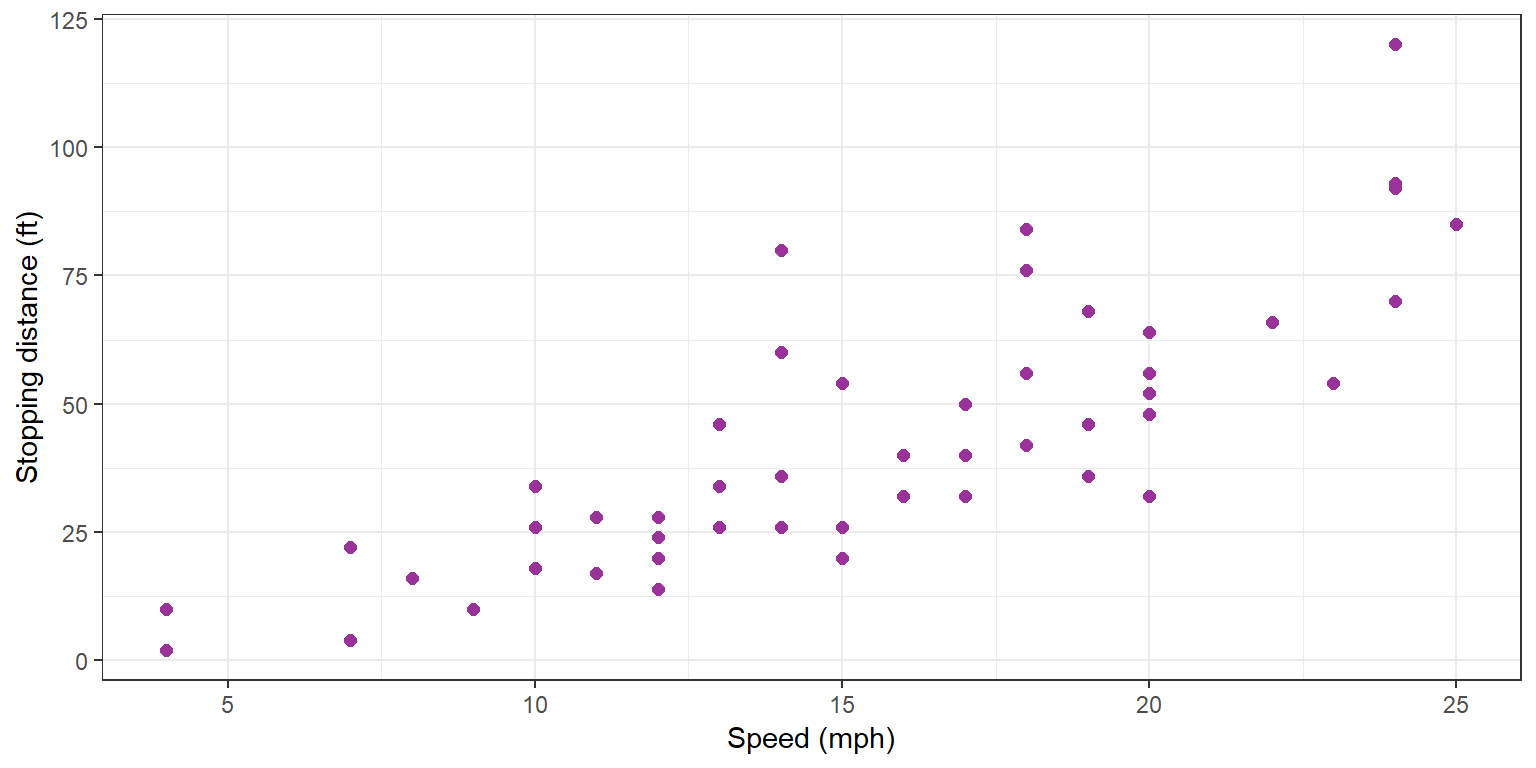The scatter plot above suggests a linearly increasing relationship between the explanatory and response variables. We are therefore assuming a linear trend which is mathematically represented by a polynomial of degree 1:

$y_j = c_0 + c_1 x_j + e_j \quad ; \quad 1 \leq j \leq n$

Let us fit this model to the data using the function lm:

attach(cars)
lm1 <- lm(dist ~ speed)

The function summary computes and returns a list of summary statistics of the fitted linear model given in lm1:

summary(lm1)
##
## Call:
## lm(formula = dist ~ speed)
##
## Residuals:
##     Min      1Q  Median      3Q     Max
## -29.069  -9.525  -2.272   9.215  43.201
##
## Coefficients:
##             Estimate Std. Error t value Pr(>|t|)
## (Intercept) -17.5791     6.7584  -2.601   0.0123 *
## speed         3.9324     0.4155   9.464 1.49e-12 ***
## ---
## Signif. codes:  0 '***' 0.001 '**' 0.01 '*' 0.05 '.' 0.1 ' ' 1
##
## Residual standard error: 15.38 on 48 degrees of freedom
## Multiple R-squared:  0.6511, Adjusted R-squared:  0.6438
## F-statistic: 89.57 on 1 and 48 DF,  p-value: 1.49e-12

Some diagnostic plots are available

par(mfrow=c(1,2))
plot(lm1, which=c(1,2))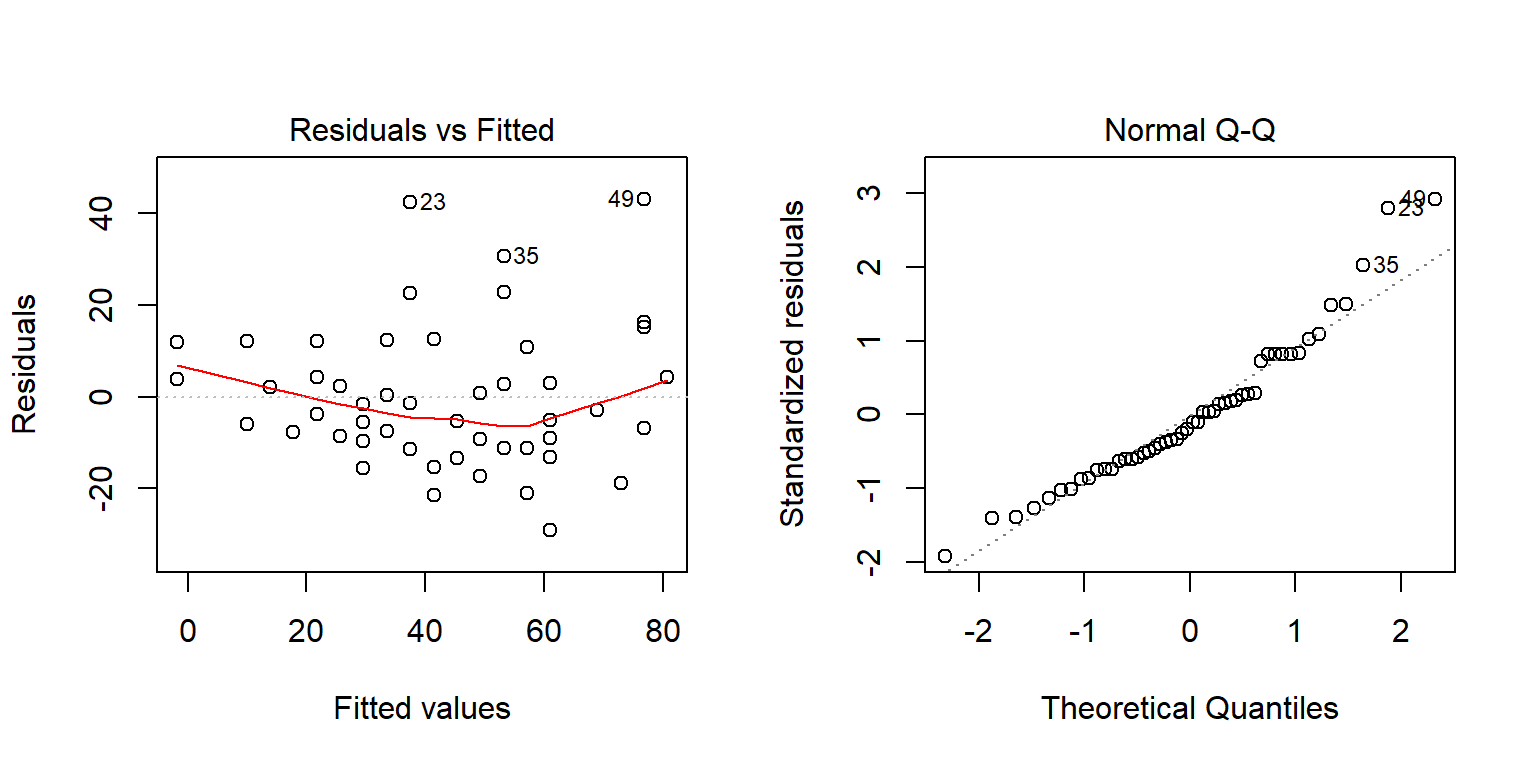as well as the log-likelihood and some information criteria:

logLik(lm1)
## 'log Lik.' -206.5784 (df=3)
AIC(lm1)
##  419.1569
BIC(lm1)
##  424.8929

Let us see what all these numbers and diagnostic plots are and how they are calculated for any polynomial model.

# 2 Fitting a polynomial model as a linear model

We need first to rewrite the polynomial regression model $y_j = c_0 + c_1 x_j + c_2 x^2_j + \ldots + c_{\degree} x^{\degree}_j + e_j$ in a matricial form $y = X\beta + e$ where $y = \left( \begin{array}{c} y_1 \\ y_2 \\ \vdots \\ y_n \end{array}\right) \quad ,\quad X = \left( \begin{array}{cccc} 1 & x_1 & \cdots & x_1^{\degree} \\ 1 & x_2 & \cdots & x_2^{\degree} \\ \vdots & \vdots & \ddots & \vdots \\ 1 & x_n & \cdots & x_n^{\degree} \end{array}\right) \quad , \quad \beta = \left( \begin{array}{c} c_0 \\ c_1 \\ \vdots \\ c_{\degree} \end{array}\right) \quad , \quad e = \left( \begin{array}{c} e_1 \\ e_2 \\ \vdots \\ e_n \end{array}\right)$ Then, $$X\beta$$ is the vector of predicted values and $$e$$ the vector of residual errors.

## 2.1 The Least squares method

A method for choosing automatically the “best parameters” $$(\hat{c}_0,\hat{c}_1)$$ consists in minimizing the sum of squared errors of prediction, i.e. the residual sum of squares (RSS) :

\begin{aligned} RSS &= \sum_{j=1}^n (y_j - f(x_j))^2 \\ &= \sum_{j=1}^n \left( y_j - \sum_{k=0}^{\degree} c_k x_j^k \right)^2 \\ &= \|y - X\beta \|^2 \end{aligned}

Then, \begin{aligned} \hat{\beta} &= \argmin{\beta}(\|y - X\beta \|^2) \\ &= (X^\prime X)^{-1}X^\prime y \end{aligned}

y <- dist
x <- speed
n <- length(y)
m <- 1
X <- NULL
for (k in (0:m))
X <- cbind(X, x^k)
beta.est <- solve(t(X)%*%X)%*%t(X)%*%y
beta.est
##            [,1]
## [1,] -17.579095
## [2,]   3.932409

## 2.2 Estimation of the residual error variance

We will assume that the residuals $$(e_j)$$ are independent random variables with mean 0 and variance $$\sigma^2$$: $\esp{e_j} = 0 \quad ; \quad \esp{e^2_j} = \sigma^2 \quad ; \quad \esp{e_j e_k} = 0 \ (j \neq k)$

Let $$\nbeta = \degree+1$$ be the length of vector $$\beta$$. Then, an unbiased estimate of $$\sigma^2$$ is \begin{aligned} \hat{\sigma}^2 &= \frac{1}{n-\nbeta} \|y - X\hat{\beta} \|^2 \\ &= \frac{1}{n-\nbeta} \sum_{j=1}^n (y_j - \hat{c}_0 - \hat{c}_1 x_j - \ldots - \hat{c}_{\degree} x_j^{\degree})^2 \end{aligned} Indeed,

\begin{aligned} \|y - X\hat{\beta} \|^2 &= \| y - X(X^\prime X)^{-1}X^\prime y\|^2 \\ &= \| \left({\rm I}_n - X(X^\prime X)^{-1}X^\prime \right) e\|^2 \\ &= e^\prime \left({\rm I}_n - X(X^\prime X)^{-1}X^\prime \right)^\prime \left({\rm I}_n - X(X^\prime X)^{-1}X^\prime \right) e \\ &= e^\prime \left({\rm I}_n - X(X^\prime X)^{-1}X^\prime \right) e \\ &= {\rm trace} \left\{ e^\prime \left({\rm I}_n - X(X^\prime X)^{-1}X^\prime \right) e \right\} \\ &= {\rm trace} \left\{ \left({\rm I}_n - X(X^\prime X)^{-1}X^\prime \right) e e^\prime \right\} \end{aligned} Then,

\begin{aligned} \esp{\|y - X\hat{\beta} \|^2} &= {\rm trace} \left( \left({\rm I}_n - X(X^\prime X)^{-1}X^\prime \right) \esp{e e^\prime} \right) \\ &= \sigma^2 {\rm trace} \left( {\rm I}_n - X(X^\prime X)^{-1}X^\prime \right) \\ &= \sigma^2 \left( {\rm trace} \left( {\rm I}_n \right) - {\rm trace} \left(X(X^\prime X)^{-1}X^\prime \right) \right) \\ &= \sigma^2 \left( n - {\rm trace} \left((X^\prime X)^{-1}X^\prime X \right) \right) \\ &= \sigma^2 \left( n - {\rm trace} \left({\rm I}_d \right) \right) \\ &= \sigma^2 ( n - d) \end{aligned}

res <- y-X%*%beta.est
d <- m+1
sigma2.est <- sum(res^2)/(n-d)
sigma2.est
##  236.5317

The standard deviation of the residual errors, called residual standard error in R, is the square root of this estimated variance

sqrt(sigma2.est)
##  15.37959

## 2.3 The standard errors of the estimates

We can remark that \begin{aligned} \hat{\beta} &= (X^\prime X)^{-1}X^\prime y \\ &= \beta + (X^\prime X)^{-1}X^\prime e \end{aligned}

Then, since $$\esp{e}=0$$, $\esp{\hat{\beta}} = \beta$

and \begin{aligned} \var{\hat\beta} &= \var{(X^\prime X)^{-1}X^\prime e} \\ &= (X^\prime X)^{-1}X^\prime \var{e} X (X^\prime X)^{-1} \\ &= \sigma^2 (X^\prime X)^{-1} \end{aligned}

We can therefore use this formula to compute the variance covariance matrix of $$(\hat{c}_0 ,\hat{c}_1)$$

V.beta <- sigma2.est*solve(t(X)%*%X)
print(V.beta)
##           [,1]       [,2]
## [1,] 45.676514 -2.6588234
## [2,] -2.658823  0.1726509

Then, the standard error of each component of $$\hat\beta$$ is defined as the square root of the diagonal elements of the variance-covariance matrix $$V=\var{\hat\beta}$$: ${\rm se}(\hat\beta_k) = \sqrt{V_{k k}}$

se.beta <- sqrt(diag(V.beta))
print(se.beta)
##  6.7584402 0.4155128

## 2.4 Statistical tests for the model parameters

We will assume that the residuals $$(e_j)$$ are independent and normally distributed with mean 0 and variance $$\sigma^2$$: $e_j \iid {\cal N}(0 \ , \ \sigma^2)$

Then, $$\hat{\beta}$$ is also normally distributed:

$\hat{\beta} \sim {\cal N}(\beta \ , \ \sigma^2 (X^\prime X)^{-1})$ and, for $$k=1, 2, \ldots , \nbeta$$, $t_k = \frac{\hat{\beta}_k - \beta_k}{{\rm se}(\hat{\beta}_k)}$ follows a $$t$$-distribution with $$n-d$$ degrees of freedom.

For each component $$\beta_k$$ of $$\beta$$, we can then perform a $$t$$-test (known as the Wald test) to test $H_{k,0} : \beta_k = 0" \quad \text{versus} \quad H_{k,1}: \beta_k \neq 0"$

Indeed, under the null hypothesis $$H_{k,0}$$, $$t_{{\rm stat}, k} = {\hat{\beta}_k}/{{\rm se}(\hat{\beta}_k)}$$ follows a $$t$$-distribution with $$n-d$$ degrees of freedom.

The $$p$$-value for this test is therefore

\begin{aligned} p_k &= \prob{|t_{n-d}| \geq |t_{{\rm stat}, k}^{\rm obs}| } \\ &= 2(1 - \prob{t_{n-d} \leq |t_{{\rm stat}, k}^{\rm obs}| } ) \end{aligned}

t.stat <- beta.est/se.beta
res.beta <- cbind(beta.est, se.beta, t.stat, 2*(1 - pt(abs(t.stat),n-d)))
colnames(res.beta) <- c("beta.est", "se.beta", "t.stat", "P(>|t|)")
res.beta
##        beta.est   se.beta    t.stat      P(>|t|)
## [1,] -17.579095 6.7584402 -2.601058 1.231882e-02
## [2,]   3.932409 0.4155128  9.463990 1.489919e-12

## 2.5 Confidence interval for the model parameters

Function confint computes confidence intervals for $$c_0$$ and $$c_1$$ (default level = 95%))

confint(lm1)
##                  2.5 %    97.5 %
## (Intercept) -31.167850 -3.990340
## speed         3.096964  4.767853

Using the fact that $$t_k$$ follows a $$t$$-distribution with $$n-\nbeta$$ degrees of freedom, we can build a confidence interval for $$\beta_k$$ of level $$1-\alpha$$:

${\rm CI}_{1-\alpha}(\beta_k) = [\hat{\beta}_k + qt_{\alpha/2, n-d}\ {\rm se}(\hat{\beta}_k) \ , \ \hat{\beta}_k + qt_{1-\alpha/2, n-d}\ {\rm se}(\hat{\beta}_k)]$ where $$qt_{\alpha/2, n-d}$$ and $$qt_{1-\alpha/2, n-d}$$ are the quantiles of order $$\alpha/2$$ and $$1-\alpha/2$$ for a $$t$$-distribution with $$n-\nbeta$$ df.

Indeed, we can easily check that $$\prob{{\rm CI}_{1-\alpha}(\beta_k) \ni \beta_k} = 1-\alpha$$.

level <- 0.95
alpha <- 1 - level
cbind(beta.est + se.beta*qt(alpha/2,n-d) , beta.est + se.beta*qt(1-alpha/2,n-d) )
##            [,1]      [,2]
## [1,] -31.167850 -3.990340
## [2,]   3.096964  4.767853

## 2.6 Coefficient of determination

The multiple R-squared $$R^2$$ is the proportion of variation in the response variable that has been explained by the model. Using the fact that $\|y - \bar{y}\|^2 = \|X\hat{\beta} - \bar{y}\|^2 + \|y - X\hat{\beta} \|^2$

\begin{aligned} R^2 &= \frac{\|X\hat{\beta} - \bar{y}\|^2}{\|y - \bar{y}\|^2} \\ &= 1 - \frac{\|y - X\hat{\beta} \|^2}{\|y - \bar{y}\|^2} \end{aligned}

1- sum((y-X%*%beta.est)^2)/sum((y-mean(y))^2)
##  0.6510794
sum((X%*%beta.est - mean(y))^2)/sum((y-mean(y))^2)
##  0.6510794

By construction, adding more predictors to the model, i.e. increasing the degree of the polynom, is always going to increase the R-squared value. Adjusted R-squared penalizes this effect by normalizing each term by the associated degree of freedom.

\begin{aligned} R^2_{\rm adj} &= 1 - \frac{\|y - X\hat{\beta} \|^2/(n-\nbeta)}{\|y - \bar{y}\|^2/(n-1)} \end{aligned} The R-squared is a purely descriptive statistics. The adjusted R-squared should be preferably used to compare the explanatory power of models built from the same dataset.

1- sum((y-X%*%beta.est)^2)/(n-d)/(sum((y-mean(y))^2)/(n-1))
##  0.6438102

## 2.7 F-test of the overall significance

A F-test is also performed to test if at least one of the coefficients $$c_1, c_2, \ldots , c_{\degree}$$ is non zero: \begin{aligned} H_0 &: \quad (c_1, c_2, \cdots ,c_{\degree}) = (0, 0, \cdots, 0) \\ H_1 &: \quad (c_1, c_2, \cdots ,c_{\degree}) \neq (0, 0, \cdots, 0) \end{aligned}

The test statistic for testing $$H_0$$ against $$H_1$$ is \begin{aligned} F_{\rm stat} &= \frac{\|X\hat{\beta} - \bar{y}\|^2/(\nbeta-1)}{\|y - X\hat{\beta} \|^2/(n-\nbeta)} \end{aligned}

F.stat <- (sum((X%*%beta.est - mean(y))^2)/(d-1))/(sum((y-X%*%beta.est)^2)/(n-d))
F.stat
##  89.56711

Let us show that, under the null hypothesis, the test statistic $$F_{\rm stat}$$ has a F distribution with $$(\nbeta-1,n-\nbeta)$$ degrees of freedom:

By construction, $$\|y - X\hat{\beta} \|^2/\sigma^2$$ has a $$\chi^2$$ distribution with $$n-d$$ df. On the other hand, under $$H_0$$, $$y_j=e_j \iid {\cal N}(0,\sigma^2)$$ and $$\|y - \bar{y}\|^2/\sigma^2$$ has a $$\chi^2$$ distribution with $$n-1$$ df.

Using the fact that $\|y - \bar{y}\|^2 = \|X\hat{\beta} - \bar{y}\|^2 + \|y - X\hat{\beta} \|^2$ We deduce that, under $$H_0$$, $$\|X\hat{\beta} - \bar{y}\|^2/\sigma^2$$ has a $$\chi^2$$ distribution with $$(n-1) - (n-d) = d-1$$ df which leads to the conclusion since $$(\chi^2(\nu_1)/\nu1)/(\chi^2(\nu_2)/\nu2) = F(\nu_1,\nu_2)$$.

The $$p$$-value of the F-test is therefore $\text{p-value(F-test)} = \prob{F_{\nbeta-1,n-\nbeta} > F_{\rm stat}}=1- \prob{F_{\nbeta-1,n-\nbeta} \leq F_{\rm stat}}$

1-pf(F.stat,d-1,n-d)
##  1.489919e-12

Remark: t-test and F-test are equivalent for linear models with only one predictor. In the case of polynomial regression of degree $$m=1$$, both tests can be used equally for testing if $$c_1=0$$. Indeed, \begin{aligned} F_{\rm stat} &= \frac{\|\hat{c}_0 + \hat{c}_1 x - \bar{y}\|^2}{\|y - \hat{c}_0 - \hat{c}_1 x\|^2/(n-2)} \\ &= \frac{\hat{c}_1^2 \|x - \bar{x}\|^2}{\hat{\sigma}^2} \\ &= \frac{\hat{c}_1^2}{se^2(\hat{c}_1)} \\ &= t_{\rm stat}^2 \end{aligned}

c(F.stat,  t.stat^2)
##  89.56711 89.56711

Furthermore, if $$t_{\rm stat}$$ has a $$t$$ distribution with $$n-2$$ df, then $$t_{\rm stat}^2$$ has a $$F$$ distribution with $$(1,n-2)$$ df. Both p-values are therefore equal.

## 2.8 The residuals

A summary of the distribution of the residuals $$y_j - \hat{f}(x_j)$$ includes the range, the mean and the quartiles:

f.est <- X%*%beta.est
summary(y-f.est)
##        V1
##  Min.   :-29.069
##  1st Qu.: -9.525
##  Median : -2.272
##  Mean   :  0.000
##  3rd Qu.:  9.215
##  Max.   : 43.201

# 3 Some diagnostic plots

Several diagnostic plots are available. The first one plots the residual versus the predicted (or fitted) values with a smooth line.

plot(lm1, which=1)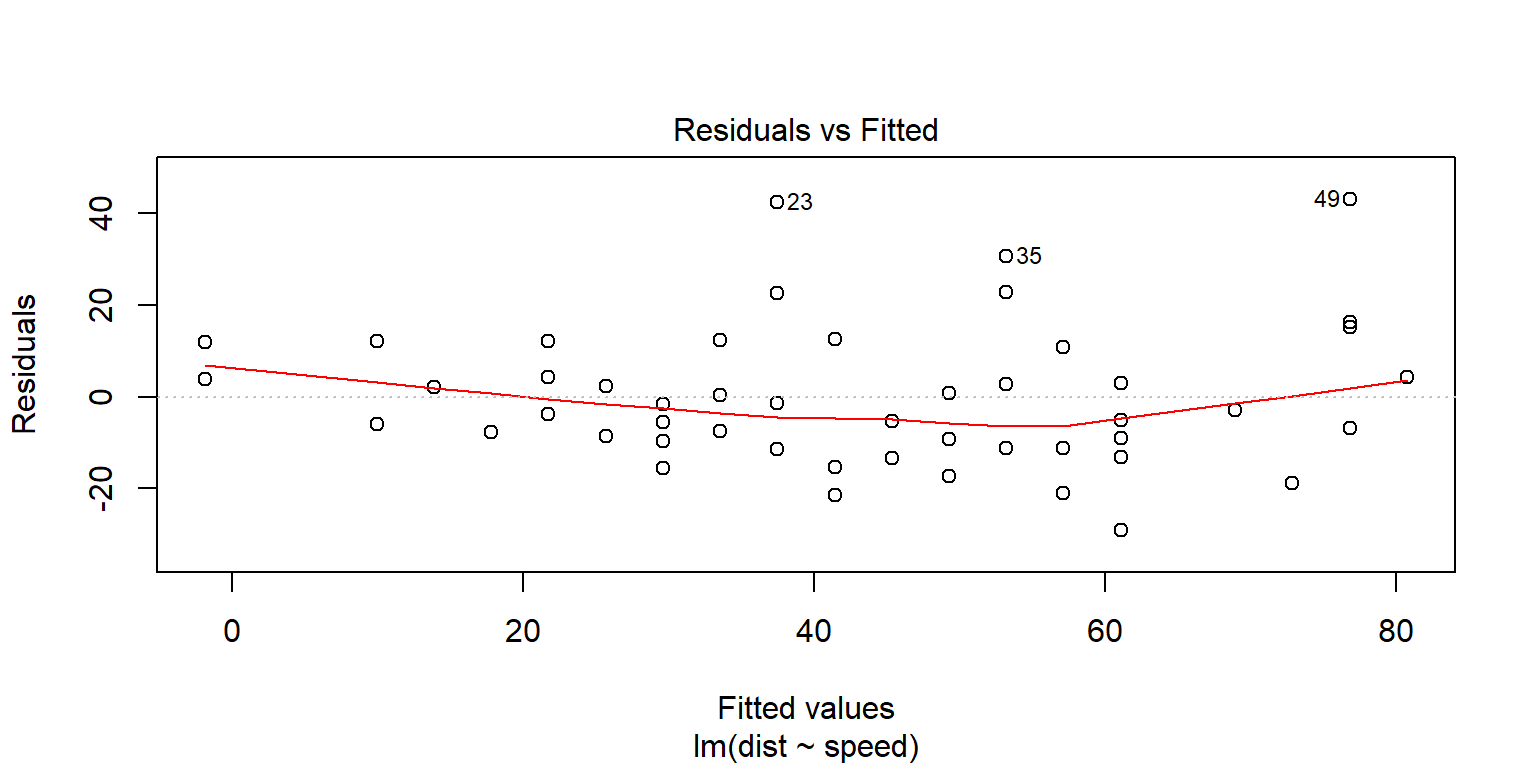We can reproduce this plot:

lo2 <- loess(resid(lm1) ~ fitted(lm1), degree = 1, span=0.8)
plot(fitted(lm1),resid(lm1))
lines(fitted(lm1),predict(lo2), col='red', lwd=2)
abline(a=0, b=0, lty=2)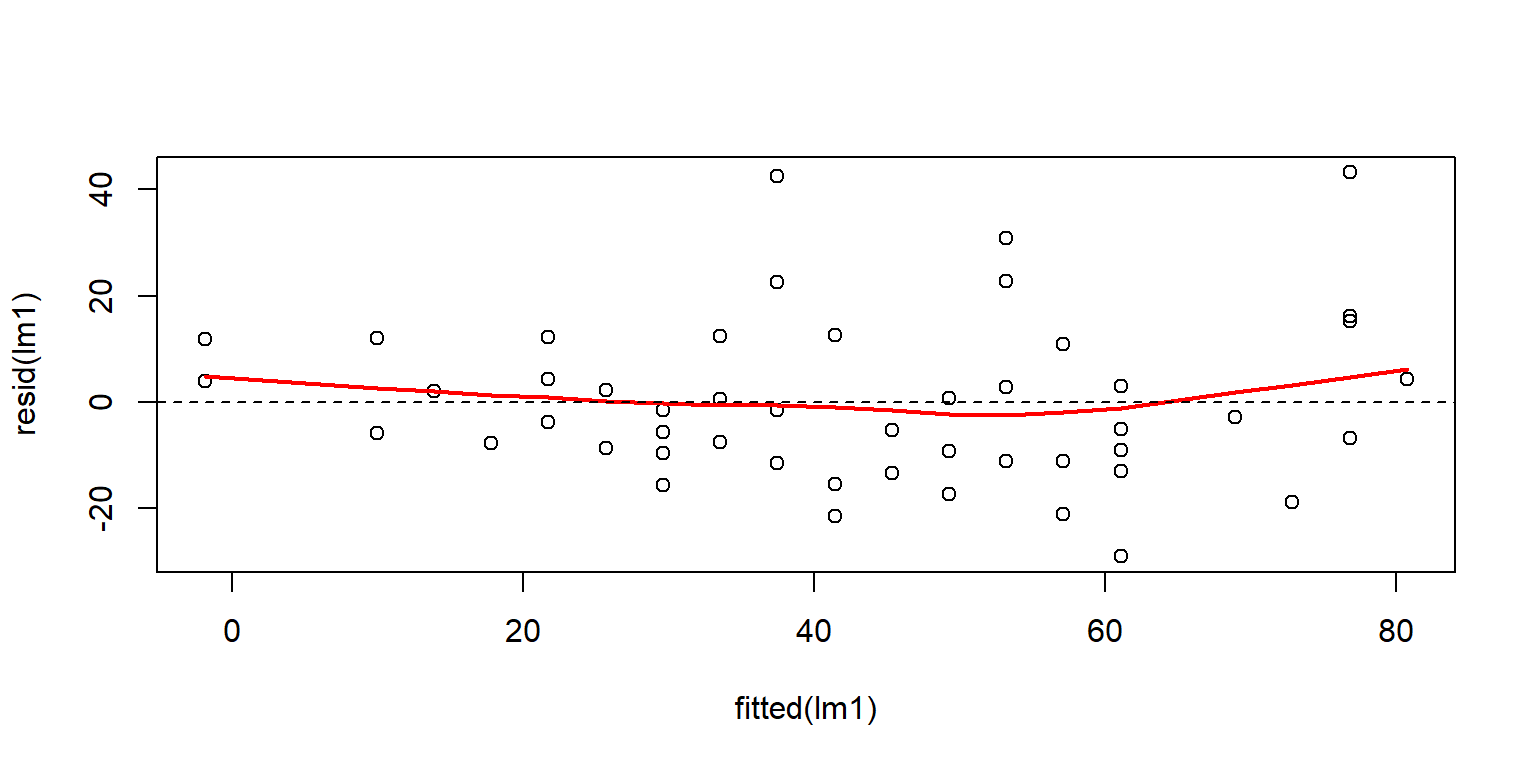The second diagnostic plot is a normal QQ plot,

plot(lm1, which=2)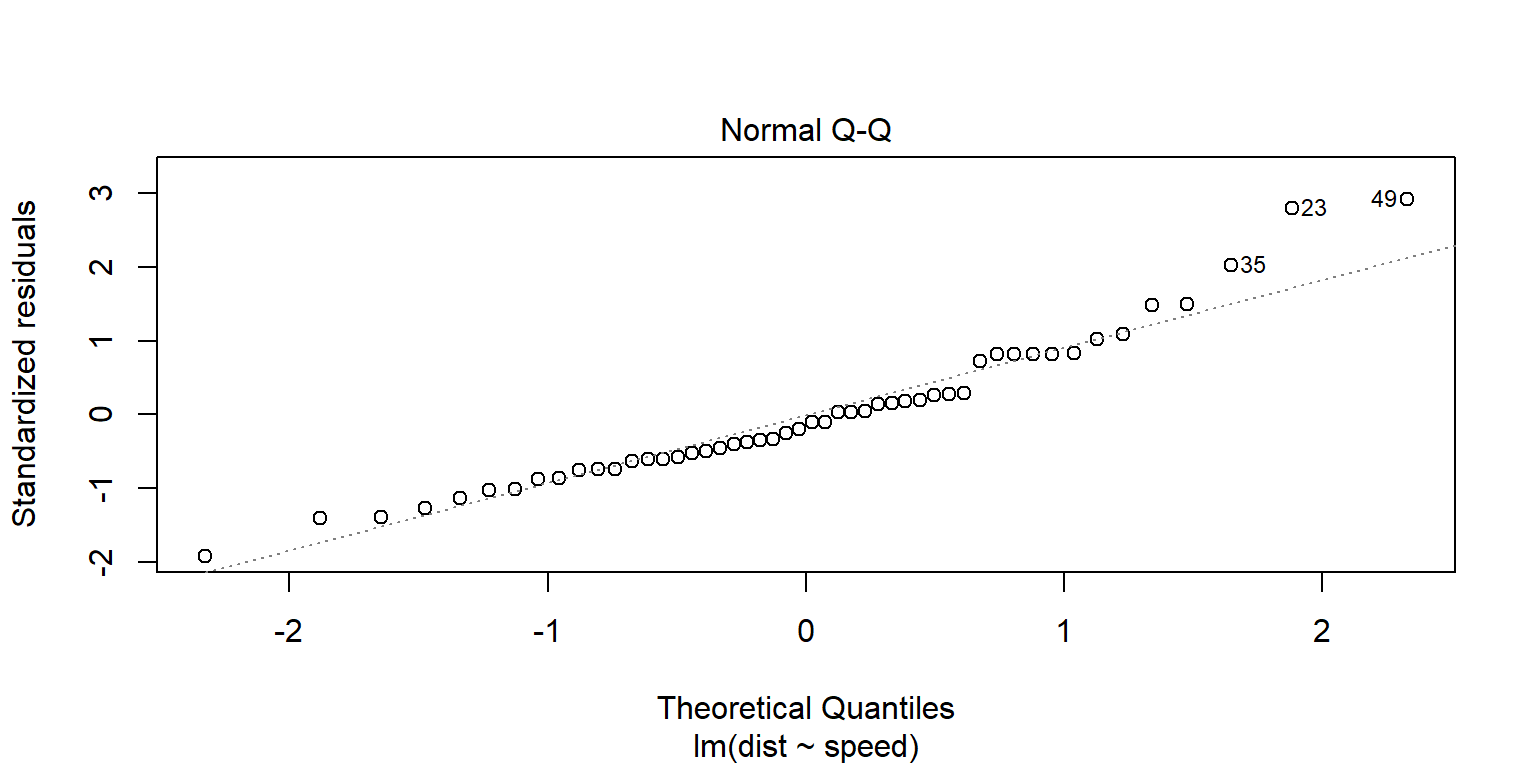The QQ plot is obtained by plotting the standardized residuals (i.e. the residuals divided by their standard deviation) versus the theoretical quantiles of order $$1/(n+1)$$, $$2/(n+1)$$, , $$n/(n+1)$$. If the residual are normally distributed, then the points should be randomly distributed around the line $$y=x$$.

plot(qnorm((